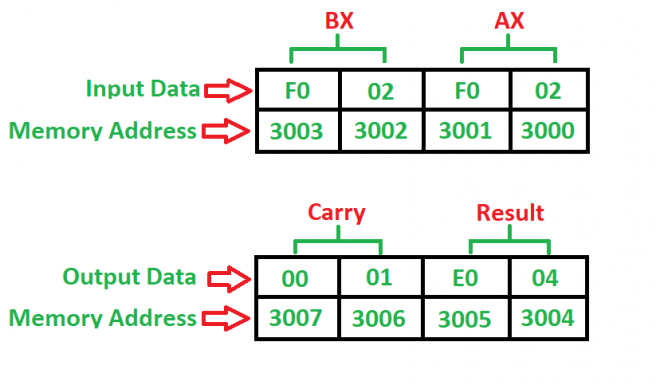# 8086 program to add two 16-bit numbers with or without carry

• Difficulty Level : Easy
• Last Updated : 22 May, 2018

Problem – Write a program to add two 16-bit numbers where starting address is 2000 and the numbers are at 3000 and 3002 memory address and store result into 3004 and 3006 memory address.

Example –Algorithm –

1. Load 0000H into CX register (for carry)
2. Load the data into AX(accumulator) from memory 3000
3. Load the data into BX register from memory 3002
4. Add BX with Accumulator AX
5. Jump if no carry
6. Increment CX by 1
7. Move data from AX(accumulator) to memory 3004
8. Move data from CX register to memory 3006
9. Stop

Program –

Memory Mnemonics Operands Comment
2000 MOV CX, 0000 [CX] <- 0000
2003 MOV AX,  [AX] <- 
2007 MOV BX,  [BX] <- 
200B ADD AX, BX [AX] <- [AX] + [BX]
200D JNC 2010 Jump if no carry
200F INC CX [CX] <- [CX] + 1
2010 MOV , AX  <- [AX]
2014 MOV , CX  <- [CX]
2018 HLT Stop

Explanation –

1. MOV is used to load and store data.
2. ADD is used to add two numbers where their one number is in accumulator or not.
3. JNC is a 2-bit command which is used to check whether the carry is generated from accumulator or not.
4. INC is used to increment an register by 1.
5. HLT is used to stop the program.
6. AX is an accumulator which is used to load and store the data.
7. BX, CX are general purpose registers where BX is used for storing second number and CX is used to store carry.
My Personal Notes arrow_drop_up
Recommended Articles
Page :